Custom Search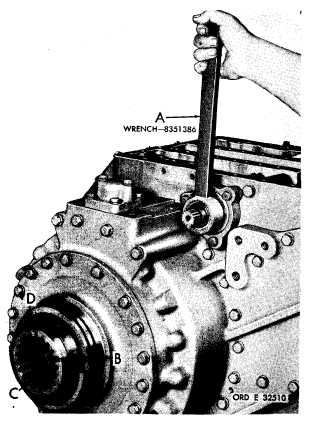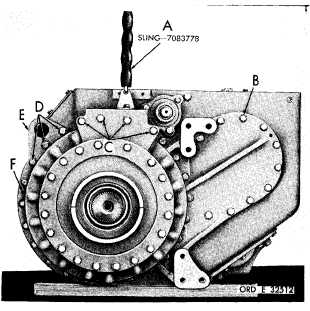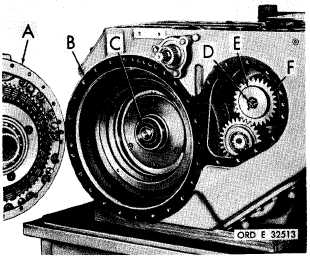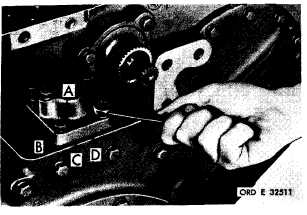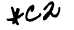P A R   75.   S T E P S   2  7 - 3 0 DlSASSEMBLY C H A P   5,   SEC   II Figure   91   (Step   27) Figure   93   (Step   29) Attach   sling   (A),   and,   using   a   5/8-inch   wrench, remove   27   bolts   (B),   four   bolts   (C)   and   three bolts   (D)   and   34   lock   washers.   Remove   lifting b r a c k e t    ( E ) .    U s e    t h r e e    b o l t s    ( B )    a s    j a c k- screws   in   holes   (F),   to   loosen   output   housing. U s i n g    w r e n c h    ( A ) ,    a p p l y    t r a n s m i s s i o n    r i g h t b r a k e . U s i n g    a    l - i n c h    w r e n c h ,    r e m o v e    b o lt (B),   lock   plate   (C)   and   output   coupling   (D). Figure   94   (Step   30) Figure   92   (Step   28) U s i n g    a    9 / 1 6 - i n c h    w r e n c h ,    r e m o v e    s i x    b o l ts (A)   and   lock   washers   (B)   retaining   accumulator body   (C).   Remove   accumulator   body   and   gas- ket   (D). Remove   right-output   housing   assembly   (A)   and g a s k e t     ( B ) .     R e m o v e     o u t     p u t     t r a n s f e r     d r i ve s h a f t    ( C ) .    U s i n g    a    1 - i n c h    w r e n c h ,    r e m o ve b o l t    ( D )    a n d    l o c k    p l a t e    ( E )    r e t a i n i n g    b r a ke coolant   oil   pump   drive   gear   (F). 9 1